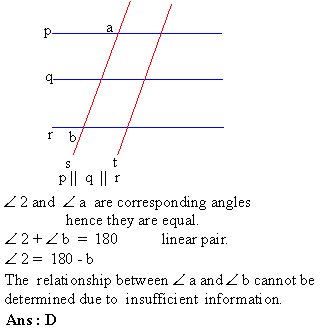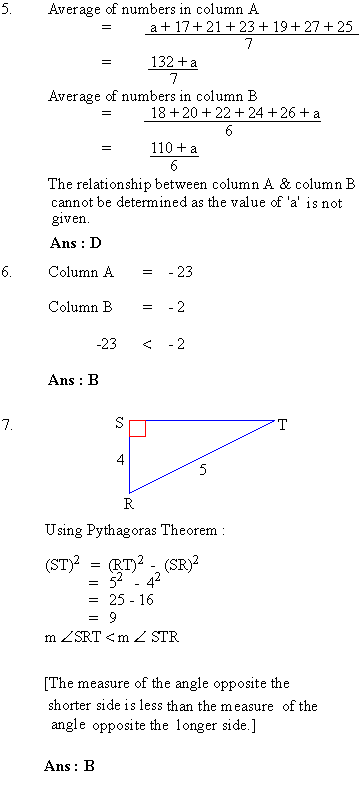Support the Monkey! Tell All your Friends and TeachersHome MonkeyNotes Printable Notes Digital Library Study Guides Study Smart Parents Tips College Planning Test Prep Fun Zone Help / FAQ How to Cite New Title Request

 Section 4 : Mathematical Reasoning Part I : Quantitative Comparison Questions The relationship between b and x is not directly given. However, the sum of two positive numbers is always going to be greater than their difference. \ b - x < b + x Ans : B An exterior angles is equal to the sum of the two remote interior angles. b = x + y Ans : C Area of circle with radius 2 = p (2)2 = 4 p Area of circle with circumference 4 p C = 4 p 2 p r = 4 p r = 2 Area of circle = p (2)2 = 4 p Ans : CIndex Test 1 Section 1 : Verbal Reasoning Section 2 : Mathematical Reasoning Section 3 : Verbal Reasoning Section 4 : Mathematical Reasoning Section 5 : Reading Comprehension Section 6 : Mathematical Reasoning Section 7 : Mathematical Reasoning Answer Key To Test 1 Answer Explanation To Test 1 Section 1 : Verbal Reasoning Section 2 : Mathematical Reasoning Section 3 : Verbal Reasoning Section 4 : Mathematical Reasoning Section 5 : Reading Comprehension Section 6 : Mathematical Reasoning Section 7 : Mathematical Reasoning Test 2
 Search: All Products Books Popular Music Classical Music Video DVD Toys & Games Electronics Software Tools & Hardware Outdoor Living Kitchen & Housewares Camera & Photo Cell Phones Keywords: